## You can use the formula A = C/4 to find the area of a circle given the circumstance. Describe another way to find the area of a circle when

Question

You can use the formula A = C/4 to find the area of a circle given the circumstance. Describe another way to find the area of a circle when given the circumstance. Help please!

in progress 0
4 months 2021-09-05T08:33:30+00:00 1 Answers 0 views 0

## Answers ( )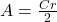Step-by-step explanation:

Given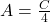Required

Describe another way to get area from circumference

Circumference C is calculated using: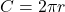Area, A is calculated using: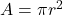Using:Divide both sides by 2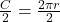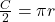Multiply both sides by r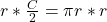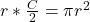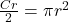The expression on the right-hand side is the area.

So, substitute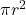for A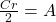Reorder